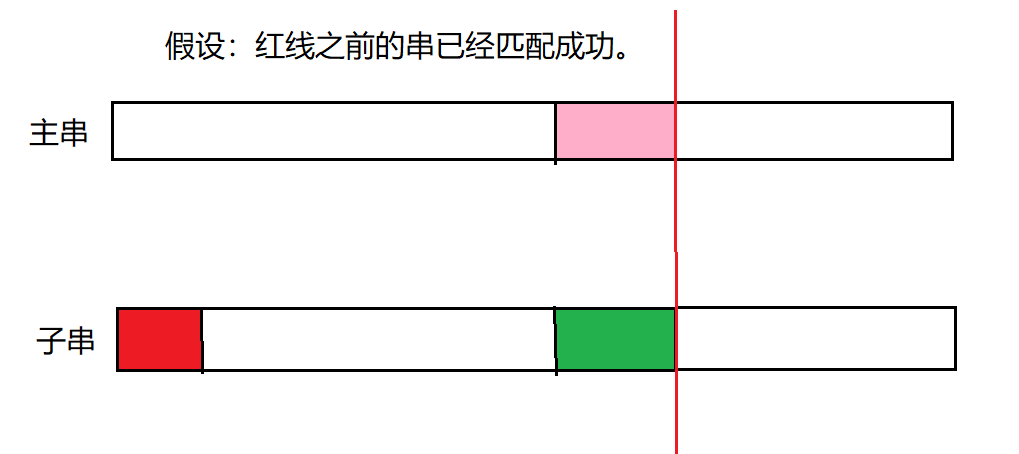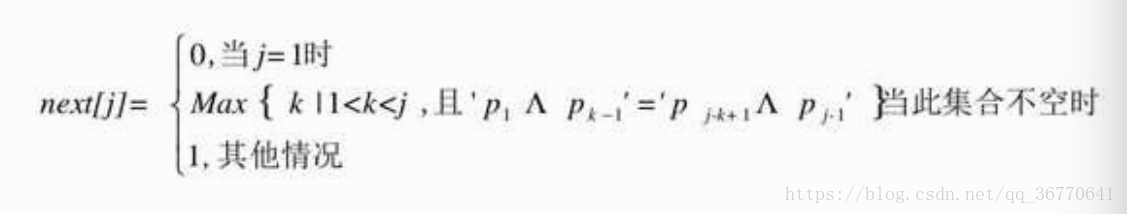# KMP--超详细的讲解

kmp 算法：无回溯的模式匹配算法，相比朴素的模式匹配算法减少了很多次没有意义的字符串比较从而使字符串的比较更加快速，高效。

## KMP 模式匹配算法原理：

1. 朴素模式匹配:（串的序号从 1 进行开始标号）
T串 a b a b e f a b
S串 a b a b a

1. i = 1 ， j = 1 时匹配成功。
2. i = 2 ， j = 2 时匹配成功。
3. i = 3 ， j = 3 时匹配成功。
4. i = 4 ， j = 4 时匹配成功。
5. i = 5 ，j = 5 时，‘e’ != ‘a’ ，匹配失败。

T串 a b a b e f a b
S串 a b a b a

T串 a b a b e f a b
S串 a b a b a

我们在第一步进行匹配时发现前面 4 个都匹配成功了，而在第 5 个的时候匹配失败了，所以要进行回溯，进行重新匹配，在回溯过程中我们发现子串中的 ‘a’ 与子串中第 2 位的 ‘d’ 是不同的，所以说，主串的第一位与子串的第一位是不可能相等的，因此，我们事先就知道匹配是不可能成功的，所以，第 2 步的比较就是多余的，我们可以去掉，当我们进行第三步匹配的时候，我们发现 S 串的前两位与 T 串相应的第 3 ，4位是匹配成功的，只有 T 串的第 5 位与 S 串的第三位是匹配失败的。有两个是成功的，那么，这个究竟为什么呐？先引入一个新的东西：

1. 串的前缀与后缀：1. NEXT数组推导：（寻找串的前后缀最大相似度）

定义：(参考大话数据结构)T = “abcdex”

j 1 2 3 4 5 6

next [j] 0 1 1 1 1 1

1）当j=1时，next=0；

2）当j=2时，j由1到j-1就只有字符“a”，属于其他情况next=1；

3）当j=3时，j由1到j-1串是“ab”，显然“a”与“b”不相等，属其他情况，next=1；

4）以后同理，所以最终此T串的next[j]为011111。
T = “abcabx”

j 1 2 3 4 5 6

next [j] 0 1 1 1 2 3

1）当j=1时，next=0；

2）当j=2时，同上例说明，next=1；

3）同理。

4）同理。

5）当j=5时，此时j由1到j-1的串是“abca”，前缀字符“a”与后缀字符“a”相等，因此可推算出k值为2（由‘p1…pk-1’=‘pj-k＋1…pj-1’，得到p1=p4）因此next=2；

6）当j=6时，j由1到j-1的串是“abcab”，由于前缀字符“ab”与后缀“ab”相等，所以next=3。

``````代码实现：
// next 数组推导：
void Get_Next(int next[], char* T) {
int i, j;
i = 1;
j = 0;
next = 0;
while (T > i) {      // T表示字符串的长度。

if (j == 0 || T[j] == T[i]) {
// T[j]表示前缀的单个字符。
++i;            // T[i]表示后缀的单个字符。
++j;
next[i] = j;

}
else                // 如果不相等，则指针退回。
j = next[j];

}
}``````

S = “aaaabcde”，T = "aaaaax"时，我们发现利用 next 数组进行匹配还是不够高效，请看下图：(后面字符省略)
1.

S串 a a a a b c d e
T串 a a a a a x

2

S串 a a a a b c d e
T串 a a a a a x

3

S串 a a a a b c d e
T串 a a a a a x

4

S串 a a a a b c d e
T串 a a a a a

5

S串 a a a a b c d e
T串 a a a a

6

S串 a a a a b c d e
T串 a a a

其next数组值分别为012345，在开始时，当i=5、j=5时，我们发现“b”与“a”不相等，如上图的 1，因此需要回溯，即：j=next=4，如图中的 2，此时“b”与第4位置的“a”依然不等，同理，j=next=3，如图中的 3，后依次是 4 5，直到j=next=0时，根据算法，此时i++、j++，得到i=6、j=1，如图中的 6。
我们发现，当中的 2 3 4 5 步骤，其实是多余的判断。由于 T 串的第二、三、四、五位置的字符都与首位的“a”相等，那么可以用首位next的值去取代与它相等的字符后续next[j]的值，这是个很好的办法。因此我们对求next函数进行了改良。

4.nextval数组推导：

j 1 2 3 4 5 6 7 8 9

next[j] 0 1 2 3 4 5 6 7 8
nextval[j] 0 0 0 0 0 0 0 0 8

1）当j=1时，nextval=0；

2）当j=2时，next值为1，第二个字符与第一个字符相等，所以nextval=nextval=0；

3）同样的道理，其后都为0……；

4）当j=9时，next值为8，第九个字符“b”与第八个字符“a”不相等，所以nextval=8。

``````代码改进：
// nextval 数组推导：
void nextval(int nextval[], char* T) {
int i, j;
i = 1;
j = 0;
nextval = 0;
while (i < T) {
if (j == 0 || T[i] == T[j]) {
++j;
++i;
if (T[i] != T[j]) {
nextval[i] = j;
}
else
nextval[i] = nextval[j];
}
else
j = nextval[j];
}
}
``````

;